Algebra 2 6-8 Guided Practice: Graphing Radical Functions
starstarstarstarstarstarstarstarstarstar
by Matthew Richardson
| 13 Questions# Solve It!# Solve It! Response & Explanation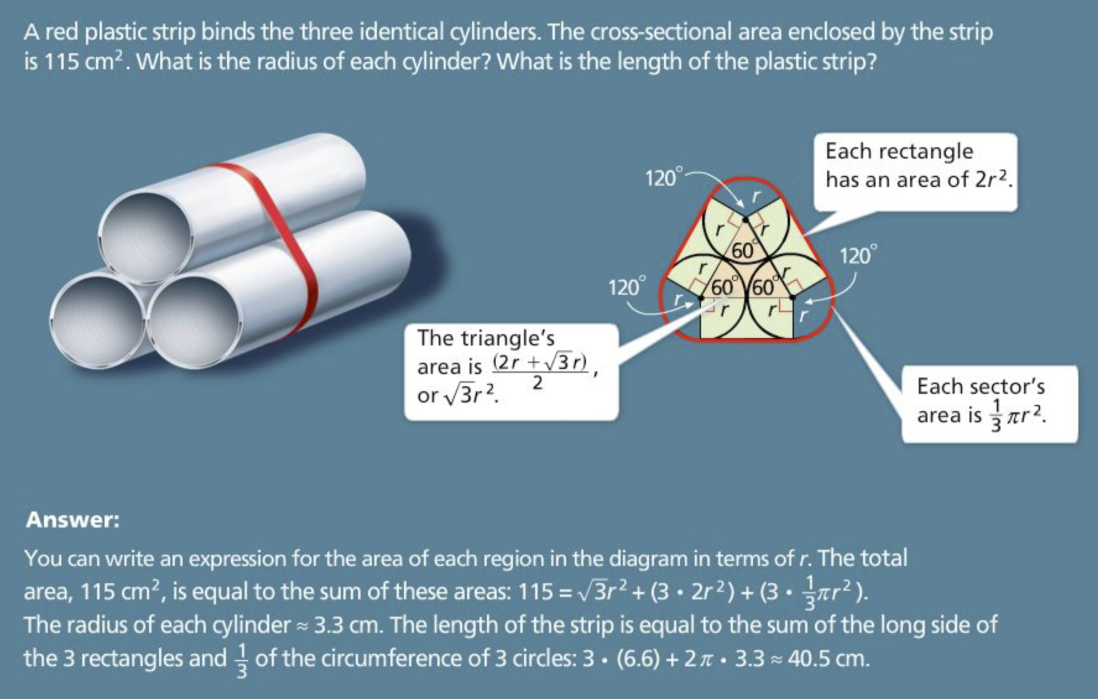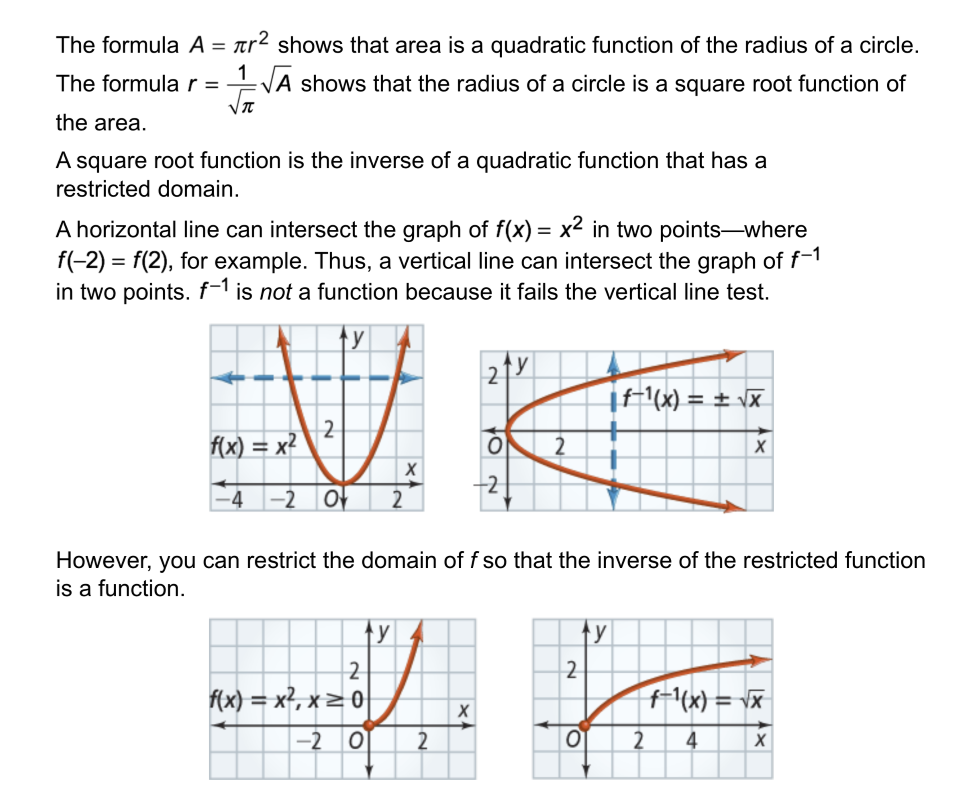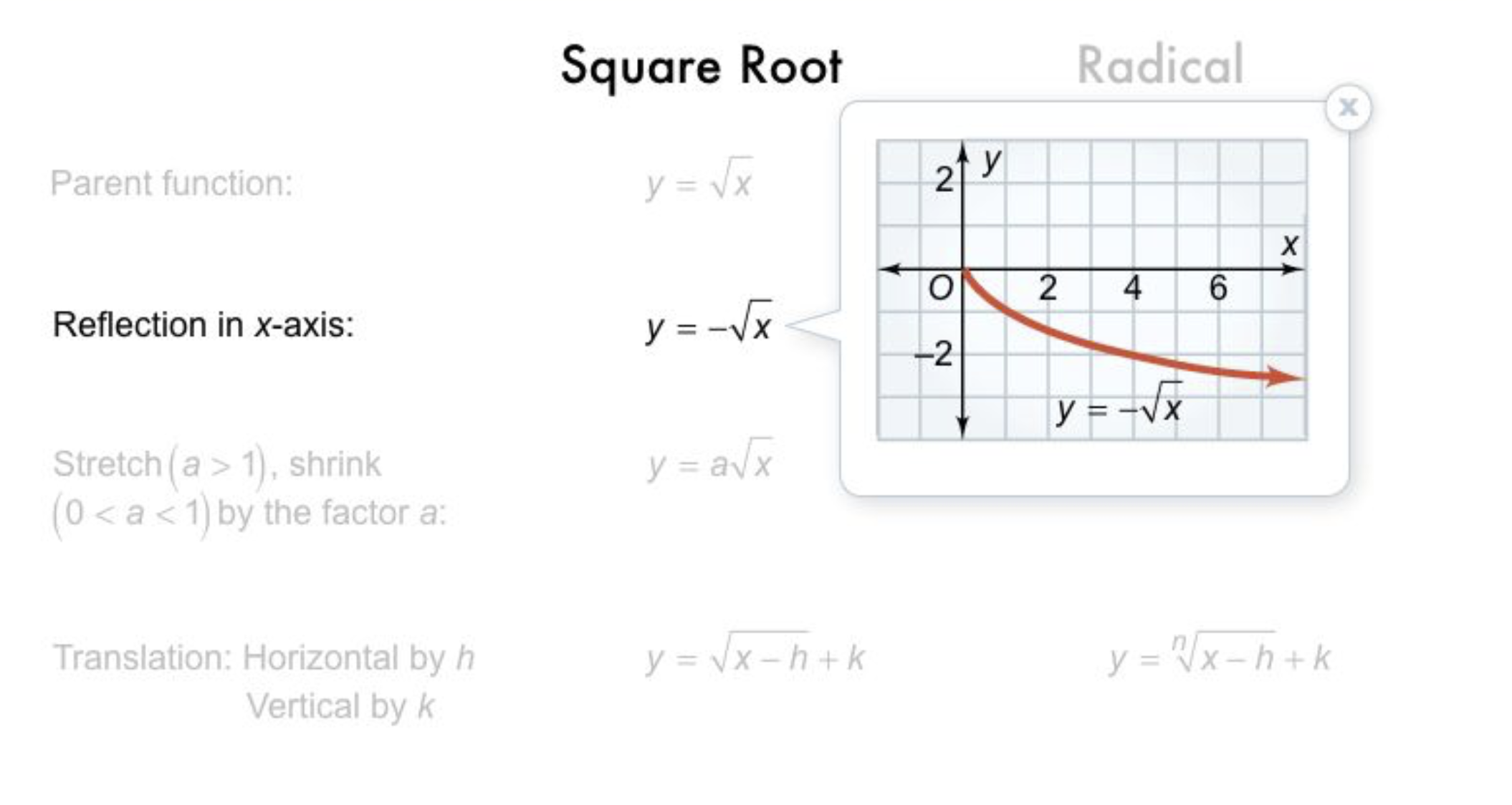a
1
2
Take Note: Take a moment to add the information about radical functions and square root functions to your notes. Don't forget to add the details from the Key Concept box about their reflections and transformations.
1
10
Take Note: What is the parent square root function ?
2
10
Take Note: What is the parent radical function ?
3
10
Problem 1 Got It?
Graph the parent square root function and the two translations in the same plane.
Notice how the translations relate to the parent function, to their graphs, and to one another.
Zoom and pan your graph to establish an appropriate viewing window.

4
10
Problem 2 Got It?
Graph the parent square root function and the two translations in the same plane.
Notice how the translations relate to the parent function, to their graphs, and to one another.
Zoom and pan your graph to establish an appropriate viewing window.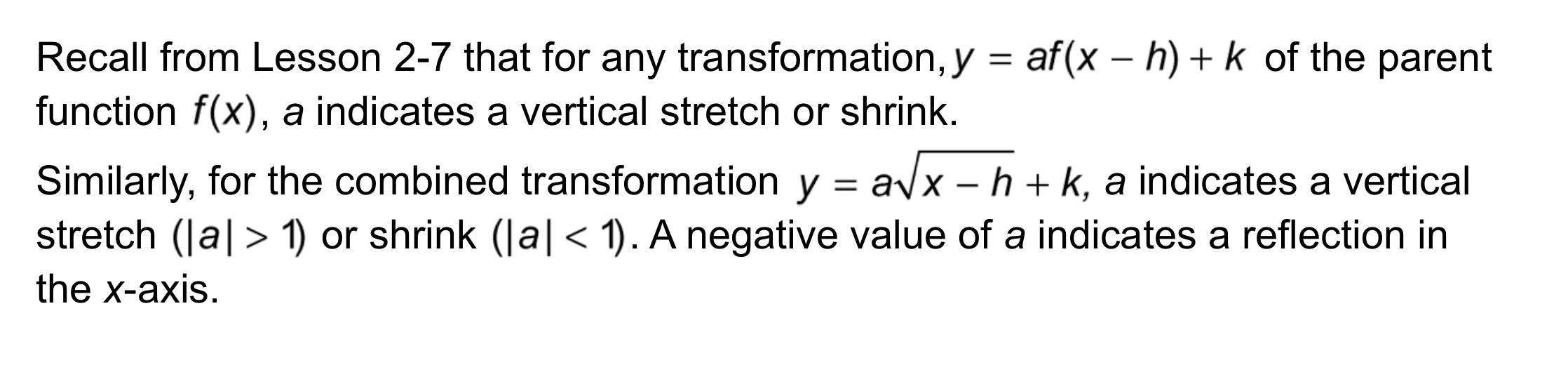a
5
6
7
Take Note: Consider the parent square root function:
and the combined transformation function:
5
10
Take Note:
What impact does a in the combined transformation function have on the graph of the parent square root function ?
6
10
Take Note:
What impact does h in the combined transformation function have on the graph of the parent square root function ?
7
10
Take Note:
What impact does k in the combined transformation function have on the graph of the parent square root function ?
8
10
Problem 3 Got It?
Graph the parent square root function and the two translations in the same plane.
Notice how the translations relate to the parent function, to their graphs, and to one another.
Zoom and pan your graph to establish an appropriate viewing window.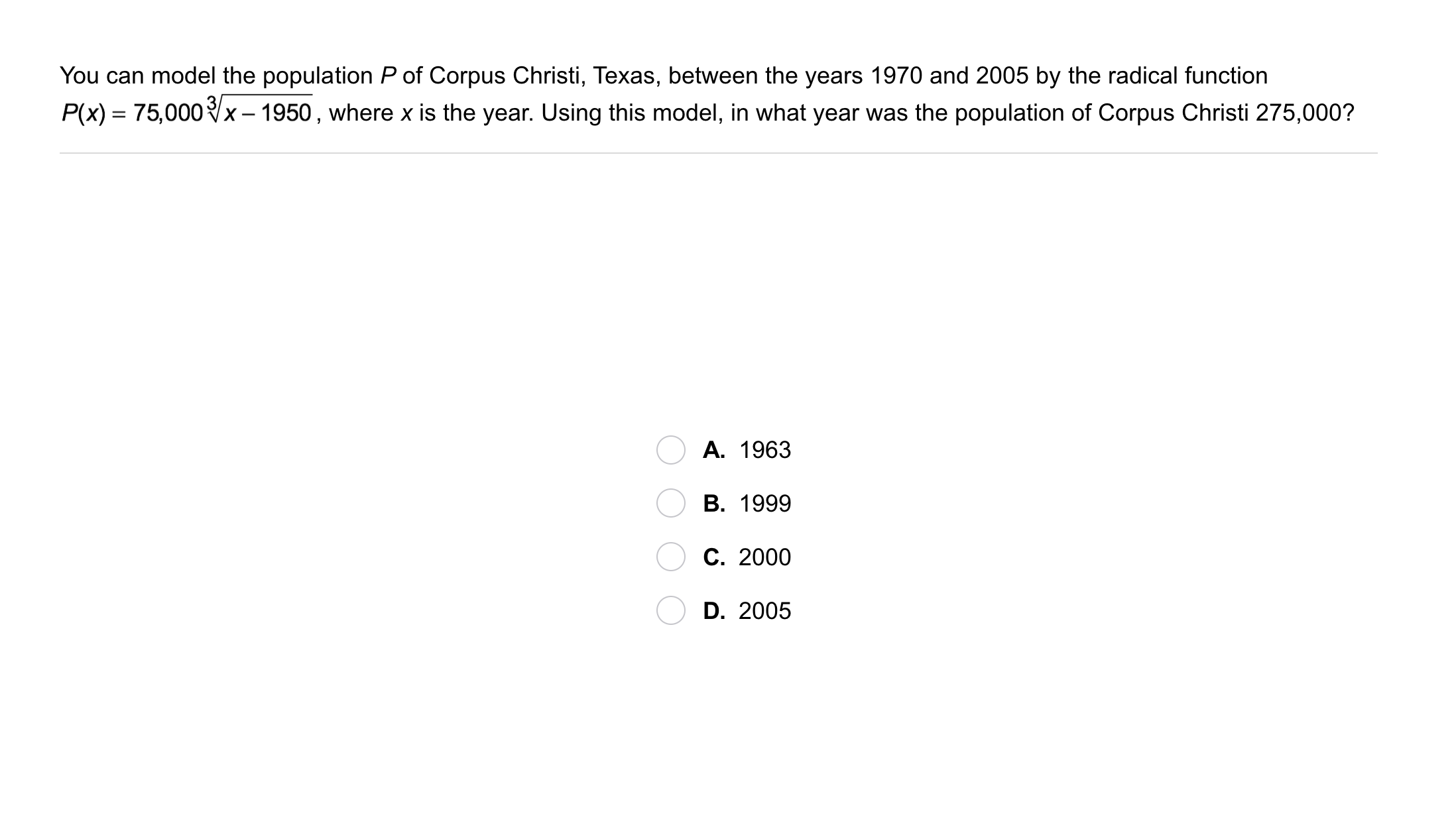9
9
10
Problem 4 Got It? You can model the population P of Corpus Christi, Texas, between the years 1970 and 2005 by the radical function below.
Using this model, in what year was the population of Corpus Christi 275,000?

The Desmos graphing utility is embedded below for your convenience.
A
B
C
D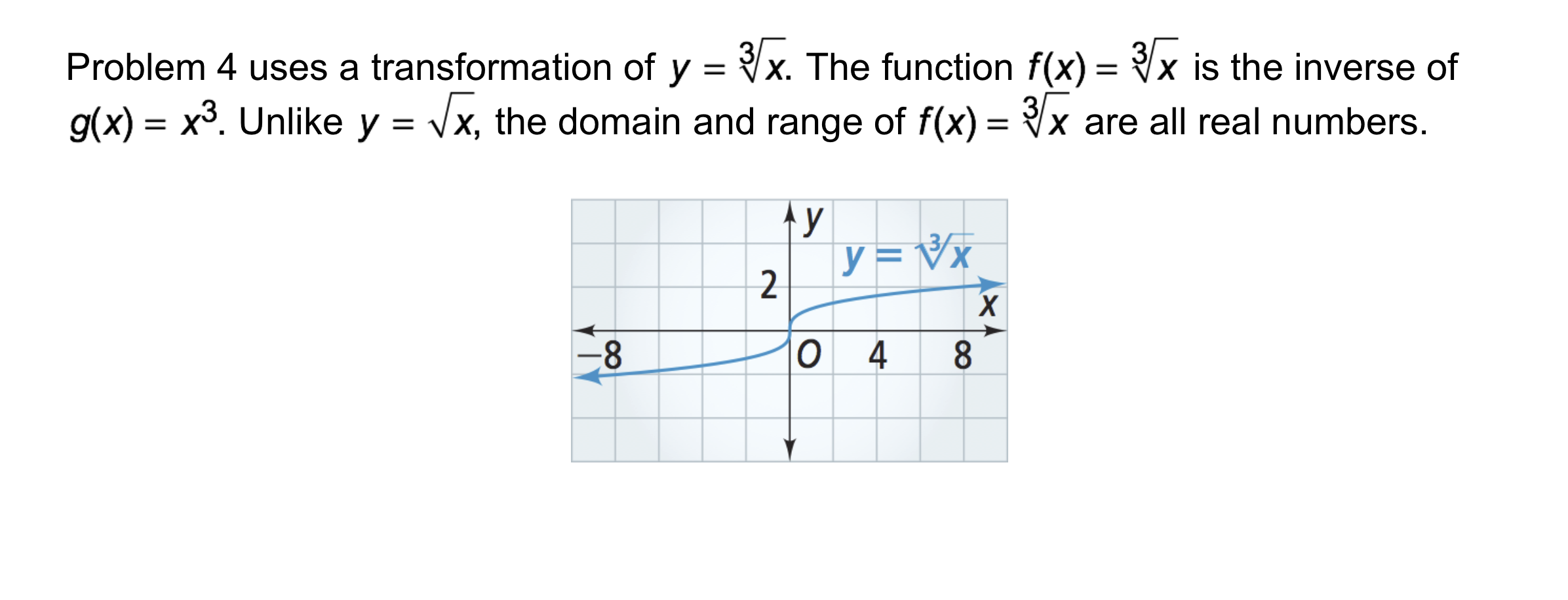10
10
Problem 5 Got It?
Graph the parent cube root function and the translation in the same plane.
Notice how the translation relates to the parent function and to its graph.
Zoom and pan your graph to establish an appropriate viewing window.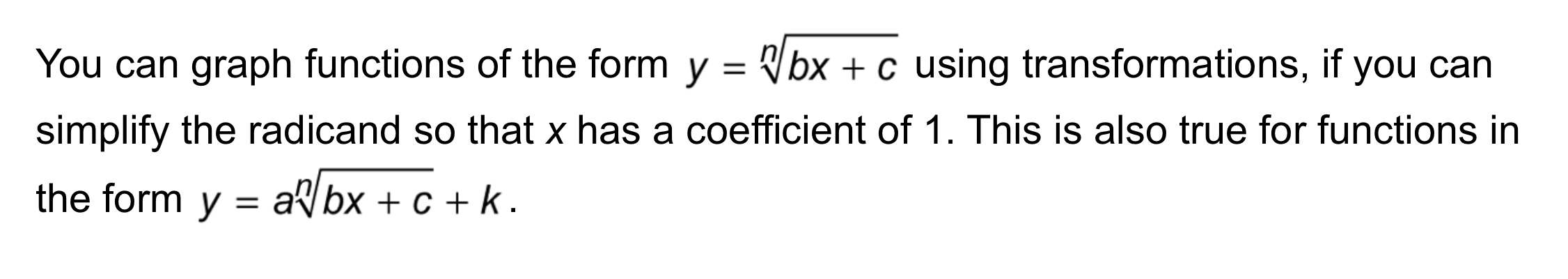11
10
Problem 6 Got It? How can you rewrite the cube root function below so that you can graph it using transformations. Describe the graph.

Stretch the graph of the parent function vertically by a factor of 2, translate 4 units left, and translate 2 units down.

Stretch the graph of the parent function vertically by a factor of 2, translate 2 units left, and translate 4 units up.

Stretch the graph of the parent function vertically by a factor of 2, translate 4 units right, and translate 2 units down.
12
10
Problem 6 Got It? Reasoning: Describe the graph of y = |9x - 18| by rewriting it in the form y = a|x - h|. How is this similar to rewriting the square root equation below (from Problem 6)?

|9x - 18| = 9|x - 2|; the graph of y = 9|x - 2| is the graph of y = |x| stretched vertically by a factor of 9 and translated right 2 units; in both cases, you are rewriting the function so that x has a coefficient of 1.
|9x - 18| = 9|x - 2|; the graph of y = 9|x - 2| is the graph of y = |x| stretched vertically by a factor of 9 and translated left 2 units; in both cases, you are rewriting the function so that x has a coefficient of 1.
|9x - 18| = 9|x - 2|; the graph of y = 9|x - 2| is the graph of y = |x| stretched vertically by a factor of 2 and translated right 9 units; in both cases, you are rewriting the function so that x has a coefficient of 1.13
13
10
Take Note: Summarize the content of this lesson.